By 岩之痕

# 一：综述

01序列的最长连续零——只知道左右区间的最长连续零，没法知道总的最长连续零

# 二：原理

（注：由于线段树的每个节点代表一个区间，以下叙述中不区分节点和区间，只是根据语境需要，选择合适的词）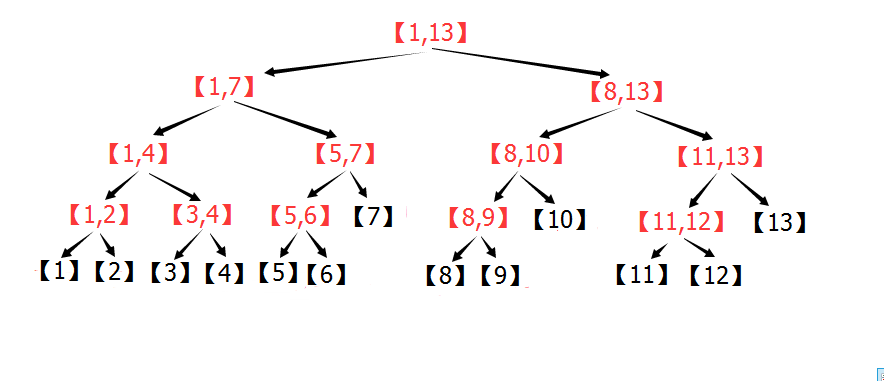## (2)线段树的区间查询：

(2.1)先给出一个粗略的证明（结合下图）：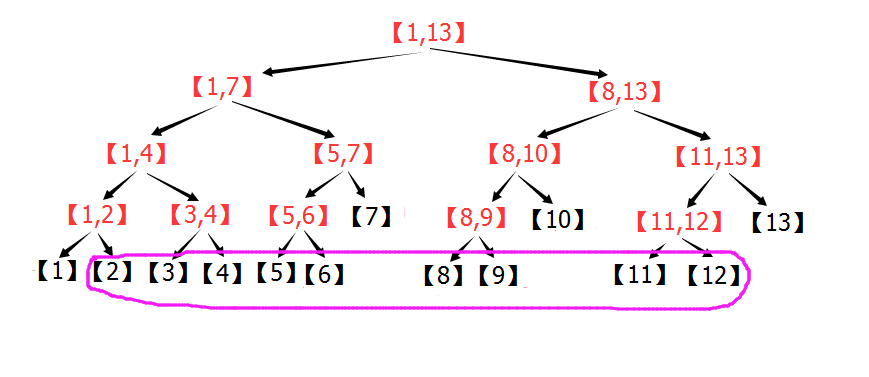(2.2)然后给出正式一点的证明：

[L,R]分成的子区间由两部分组成：

[L,R]一共被分成了个区间。n=3,4时，有很多组区间的分解可以达到最小上界。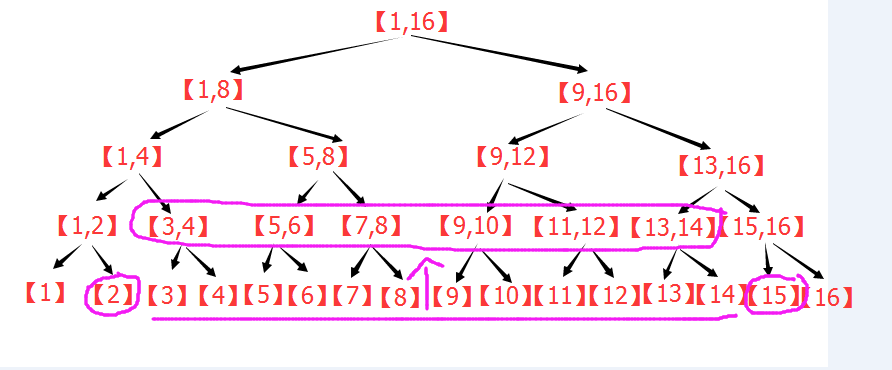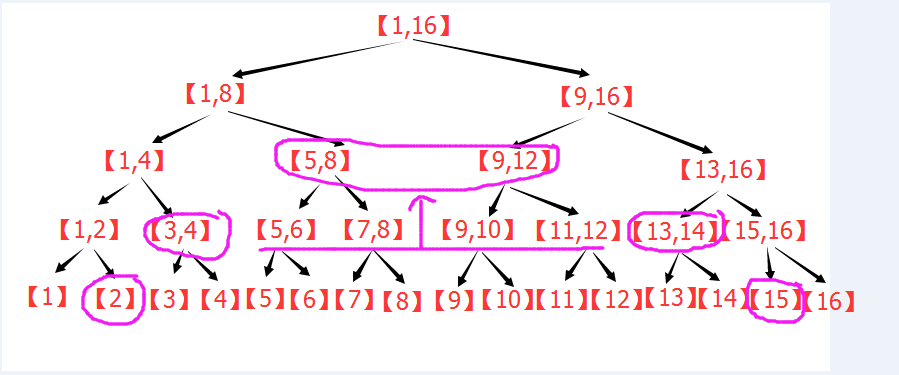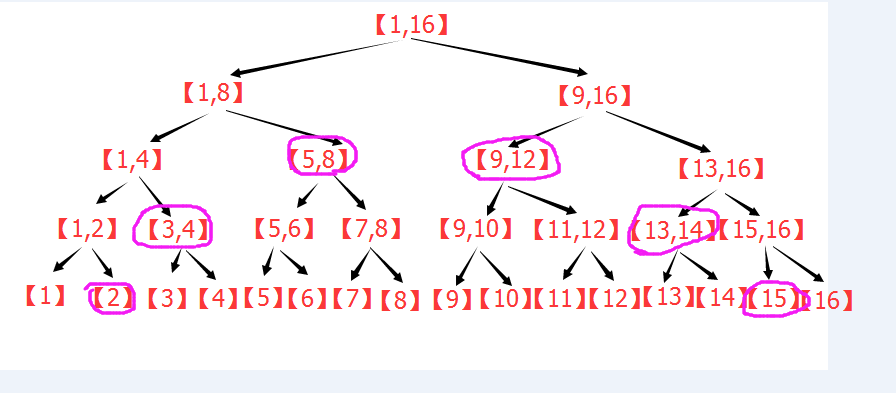## (3)线段树的区间修改：

注意：如果区间修改时不下推标记，那么PushUp函数中，必须考虑本节点的标记。
而如果所有操作都下推标记，那么PushUp函数可以不考虑本节点的标记，因为本节点的标记一定已经被下推了（也就是对本节点无效了）

## (4)线段树的存储结构：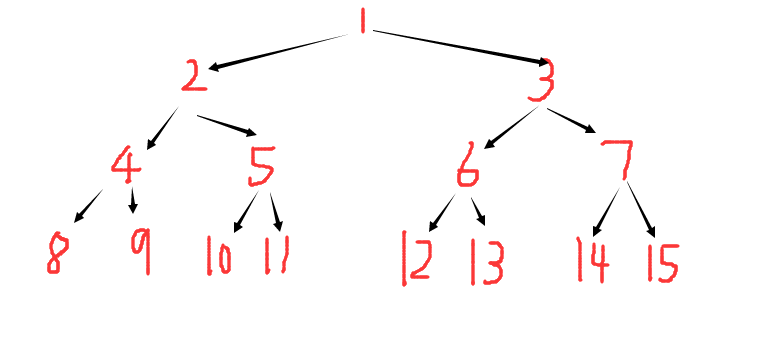# 三：递归实现

(0)定义：

#define maxn 100007  //元素总个数
#define ls l,m,rt<<1
#define rs m+1,r,rt<<1|1
int A[maxn],n;//存原数组数据下标[1,n] 

(1)建树：

//PushUp函数更新节点信息 ，这里是求和
void PushUp(int rt){Sum[rt]=Sum[rt<<1]+Sum[rt<<1|1];}
//Build函数建树
void Build(int l,int r,int rt){ //l,r表示当前节点区间，rt表示当前节点编号
if(l==r) {//若到达叶节点
Sum[rt]=A[l];//储存数组值
return;
}
int m=(l+r)>>1;
//左右递归
Build(l,m,rt<<1);
Build(m+1,r,rt<<1|1);
//更新信息
PushUp(rt);
}

(2)点修改：

void Update(int L,int C,int l,int r,int rt){//l,r表示当前节点区间，rt表示当前节点编号
if(l==r){//到叶节点，修改
Sum[rt]+=C;
return;
}
int m=(l+r)>>1;
//根据条件判断往左子树调用还是往右
if(L <= m) Update(L,C,l,m,rt<<1);
else       Update(L,C,m+1,r,rt<<1|1);
PushUp(rt);//子节点更新了，所以本节点也需要更新信息
} 

(3)区间修改：

void Update(int L,int R,int C,int l,int r,int rt){//L,R表示操作区间，l,r表示当前节点区间，rt表示当前节点编号
if(L <= l && r <= R){//如果本区间完全在操作区间[L,R]以内
Sum[rt]+=C*(r-l+1);//更新数字和，向上保持正确
return ;
}
int m=(l+r)>>1;
PushDown(rt,m-l+1,r-m);//下推标记
//这里判断左右子树跟[L,R]有无交集，有交集才递归
if(L <= m) Update(L,R,C,l,m,rt<<1);
if(R >  m) Update(L,R,C,m+1,r,rt<<1|1);
PushUp(rt);//更新本节点信息
} 

(4)区间查询：

void PushDown(int rt,int ln,int rn){
//ln,rn为左子树，右子树的数字数量。
//下推标记
//清除本节点标记
}
}

int Query(int L,int R,int l,int r,int rt){//L,R表示操作区间，l,r表示当前节点区间，rt表示当前节点编号
if(L <= l && r <= R){
//在区间内，直接返回
return Sum[rt];
}
int m=(l+r)>>1;
//下推标记，否则Sum可能不正确
PushDown(rt,m-l+1,r-m);

//累计答案
int ANS=0;
if(L <= m) ANS+=Query(L,R,l,m,rt<<1);
if(R >  m) ANS+=Query(L,R,m+1,r,rt<<1|1);
return ANS;
} 

(5)函数调用:


//建树
Build(1,n,1);
//点修改
Update(L,C,1,n,1);
//区间修改
Update(L,R,C,1,n,1);
//区间查询
int ANS=Query(L,R,1,n,1);

# 四：非递归原理

## 点修改：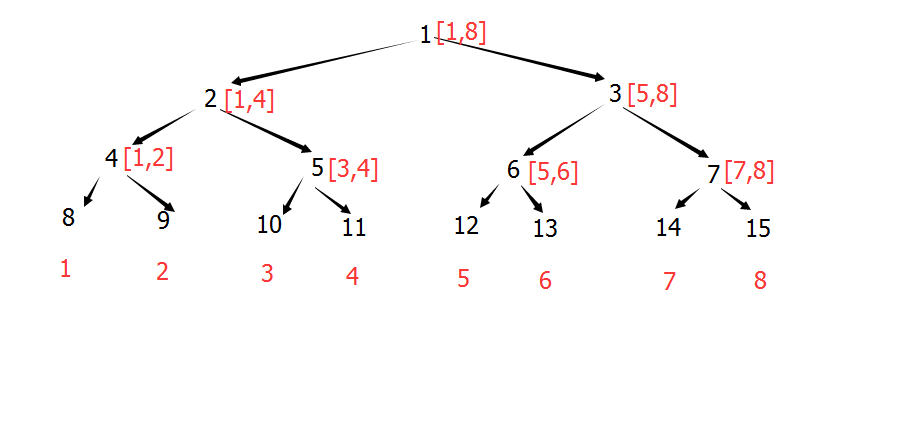【在上面的图片中，红色为原数组下标，黑色为存储下标】

## 点修改下的区间查询：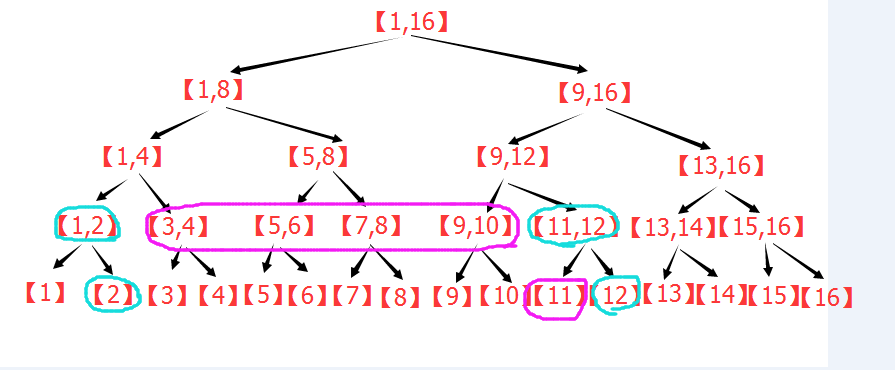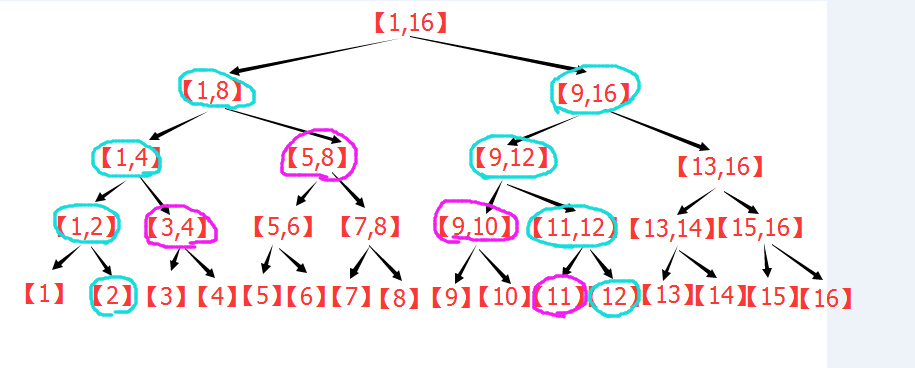## 区间修改下的区间查询：# 五：非递归实现

(0)定义

//
#define maxn 100007
int A[maxn],n,N;//原数组,n为原数组元素个数 ,N为扩充元素个数
int Sum[maxn<<2];//区间和
int Add[maxn<<2];//懒惰标记 

(1)建树:

//
void Build(int n){
//计算N的值
N=1;while(N < n+2) N <<= 1;
//更新叶节点
for(int i=1;i<=n;++i) Sum[N+i]=A[i];//原数组下标+N=存储下标
//更新非叶节点
for(int i=N-1;i>0;--i){
//更新所有非叶节点的统计信息
Sum[i]=Sum[i<<1]+Sum[i<<1|1];
}
} 

(2)点修改：

A[L]+=C
//
void Update(int L,int C){
for(int s=N+L;s;s>>=1){
Sum[s]+=C;
}
} 

(3)点修改下的区间查询：

s和t分别代表之前的论述中的左右蓝色节点，其余的代码根据之前的论述应该很容易看懂了。
s^t^1 在s和t的父亲相同时值为0，终止循环。

//
int Query(int L,int R){
int ANS=0;
for(int s=N+L-1,t=N+R+1;s^t^1;s>>=1,t>>=1){
if(~s&1) ANS+=Sum[s^1];
if( t&1) ANS+=Sum[t^1];
}
return ANS;
} 

(4)区间修改：

A[L..R]+=C
<span style="font-size:14px;">//
void Update(int L,int R,int C){
int s,t,Ln=0,Rn=0,x=1;
//Ln:  s一路走来已经包含了几个数
//Rn:  t一路走来已经包含了几个数
//x:   本层每个节点包含几个数
for(s=N+L-1,t=N+R+1;s^t^1;s>>=1,t>>=1,x<<=1){
//更新Sum
Sum[s]+=C*Ln;
Sum[t]+=C*Rn;
}
//更新上层Sum
for(;s;s>>=1,t>>=1){
Sum[s]+=C*Ln;
Sum[t]+=C*Rn;
}
} </span>

(5)区间修改下的区间查询：

//
int Query(int L,int R){
int s,t,Ln=0,Rn=0,x=1;
int ANS=0;
for(s=N+L-1,t=N+R+1;s^t^1;s>>=1,t>>=1,x<<=1){
//根据标记更新
//常规求和
if(~s&1) ANS+=Sum[s^1],Ln+=x;
if( t&1) ANS+=Sum[t^1],Rn+=x;
}
//处理上层标记
for(;s;s>>=1,t>>=1){
}
return ANS;
}

# 六：线段树解题模型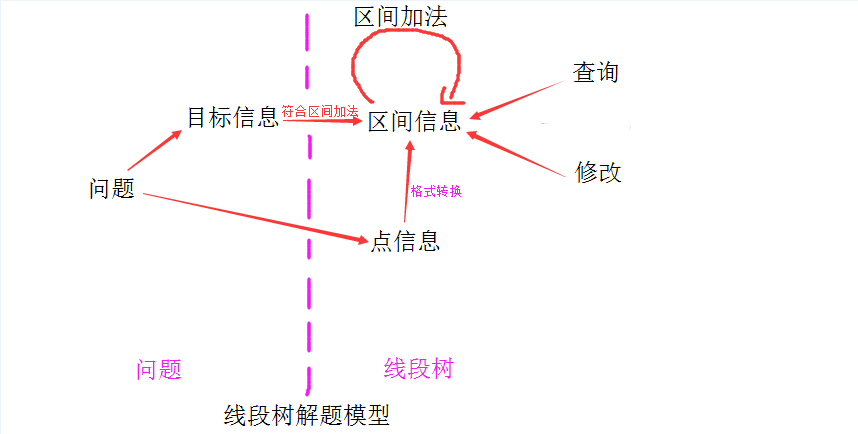--------统计信息：统计节点代表的区间的信息，一般自下而上更新
--------标记：对操作进行标记（在区间修改时需要），一般自上而下传递，或者不传递

1.区间加法
2.建树，点信息到区间信息的转换
3.每种操作（包括查询，修改）对区间信息的调用，修改

## （1）：字符串哈希

1.区间加法 ：（PushUp函数,Pow[a]=K^a）
2.点信息->区间信息：(叶节点上，区间只包含一个点，所以需要将点信息转换成区间信息)

//
#define K 137
#define maxn 100001
char str[maxn];
int Pow[maxn];//K的各个次方
struct Node{
int KeyL,KeyR;
Node():KeyL(0),KeyR(0){}
void init(){KeyL=KeyR=0;}
}node[maxn<<2];
void PushUp(int L,int R,int rt){
node[rt].KeyL=node[rt<<1].KeyL+node[rt<<1|1].KeyL*Pow[L];
node[rt].KeyR=node[rt<<1].KeyR*Pow[R]+node[rt<<1|1].KeyR;
}

## （2）：最长连续零

1.区间加法：

-（1）：左子区间最长连续零
-（2）：右子区间最长连续零
-（3）：左右子区间拼起来，而在中间生成的连续零（可能长于两个子区间的最长连续零）

--（1）：左区间不是全零，那么等于左区间的左最长连续零
--（2）：左区间全零，那么等于左区间0的个数加上右区间的左最长连续零

2.点信息->区间信息 ：

//
#define maxn 200001
using namespace std;
int L[maxn<<2];//从左开始连续零个数
int R[maxn<<2];//从右
int Max[maxn<<2];//区间最大连续零
bool Pure[maxn<<2];//是否全零
int M;
void PushUp(int rt,int k){//更新rt节点的四个数据  k来选择两棵线段树
Pure[rt][k]=Pure[rt<<1][k]&&Pure[rt<<1|1][k];
Max[rt][k]=max(R[rt<<1][k]+L[rt<<1|1][k],max(Max[rt<<1][k],Max[rt<<1|1][k]));
L[rt][k]=Pure[rt<<1][k]?L[rt<<1][k]+L[rt<<1|1][k]:L[rt<<1][k];
R[rt][k]=Pure[rt<<1|1][k]?R[rt<<1|1][k]+R[rt<<1][k]:R[rt<<1|1][k];
}



## （3）：计数排序

1.区间加法

2.点信息->区间信息：把对应字符的数量设置成1，其余为0，排序标记为false。

3.标记下推

4.区间修改

//
struct Node{
int d;//计数排序
int D;//总数
bool sorted;//是否排好序
bool Inc;//是否升序
};

## （4）总结：

：将问题转换成点信息目标信息

：将目标信息根据需要扩充成区间信息
1.增加信息符合区间加法。
2.增加标记支持区间操作。

：代码中的主要模块：
1.区间加法
2.标记下推
3.点信息->区间信息
4.操作（各种操作，包括修改和查询）

# 七：扫描线

POJ 1177 Picture:给定若干矩形求合并之后的图形周长    题解
HDU 1255 覆盖的面积：给定平面上若干矩形,求出被这些矩形覆盖过至少两次的区域的面积.   题解
HDU 3642 Get The Treasury：给定若干空间立方体，求重叠了3次或以上的体积（这个是扫描面，每个面再扫描线）题解

POJ 2482 Stars in your window : 给定一些星星的位置和亮度，求用W*H的矩形能够框住的星星亮度之和最大为多少。

## 扫描线求重叠矩形面积：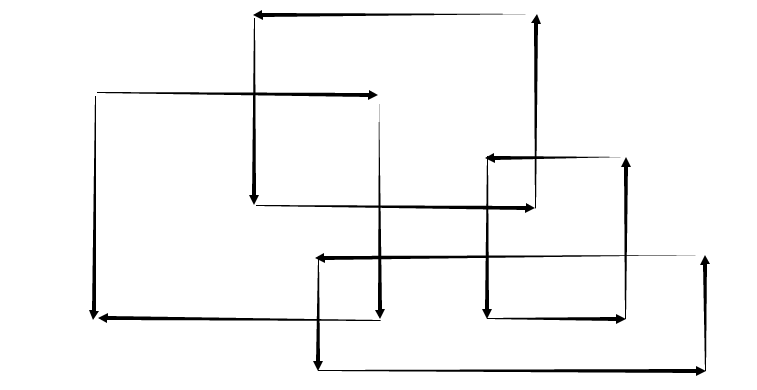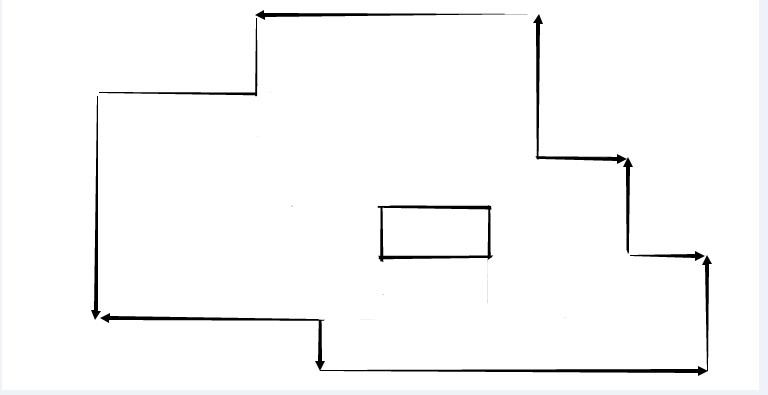X1:首先遇到X1,将第一条线段加入线段树，由线段树统计得到线段长度为L1.

X2:然后继续扫描到X2,此时要进行两个动作：
1.计算面积，目前扫过的面积=L1*(X2-X1)
2.更新线段。由于X2处仍然是入边，所以往线段树中又加了一条线段，加的这条线段可以参考3幅图中的第一幅。

X3:继续扫描到X3，步骤同X2

X4:扫描到X4有些不一样了。

//
struct LINE{
int x;//横坐标
int y1,y2;//矩形纵向线段的左右端点
bool In;//标记是入边还是出边
bool operator < (const Line &B)const{return x < B.x;}
}Line[maxn]; 

//
int PreL=0;//前一个L值,刚开始是0，所以第一次计算时不会引入误差
int X;//X值
int ANS=0;//存累计面积
int I=0;//线段的下标

while(I < Ln){
//先计算面积
ANS+=PreL*(Line[I].x-X);
X=Line[I].x;//更新X值
//对所有X相同的线段进行操作
while(I < Ln && Line[I].x == X){
//根据入边还是出边来选择加入线段还是移除线段
if(Line[I].In) Cover(Line[I].y1,Line[I].y2-1,1,n,1);
else         Uncover(Line[I].y1,Line[I].y2-1,1,n,1);
++I;
}
}

## 需要解决的几个问题：

（1）：线段树进行线段操作时，每个点的含义（比如为什么Cover函数中，y2后面要-1）。
（2）：线段树如何维护扫描线过程中的覆盖线段长度。
（3）：线段树如何维护扫描线过程中线段的数量。

### （1）：线段树中点的含义

//
int Rank[maxn],Rn;
void SetRank(){//调用前，所有y值被无序存入Rank数组，下标为[1..Rn]
int I=1;
//第一步排序
sort(Rank+1,Rank+1+Rn);
//第二步去除重复值
for(int i=2;i<=Rn;++i) if(Rank[i]!=Rank[i-1]) Rank[++I]=Rank[i];
Rn=I;
//此时，所有y值被从小到大无重复地存入Rank数组，下标为[1..Rn]
}
int GetRank(int x){//给定x，求x的下标
//二分法求下标
int L=1,R=Rn,M;//[L,R] first >=x
while(L!=R){
M=(L+R)>>1;
if(Rank[M]<x) L=M+1;
else R=M;
}
return L;
}

//
if(Line[I].In) Cover(GetRank(Line[I].y1),GetRank(Line[I].y2)-1,1,n,1);
else         Uncover(GetRank(Line[I].y1),GetRank(Line[I].y2)-1,1,n,1);

### （2）：如何维护覆盖线段长度

//
struct Node{
int Cover;//区间整体被覆盖的次数
int L;//Length : 所代表的区间总长度
int CL;//Cover Length :实际覆盖长度
Node operator +(const Node &B)const{
Node X;
X.Cover=0;//因为若上级的Cover不为0，不会调用子区间加法函数
X.L=L+B.L;
X.CL=CL+B.CL;
return X;
}
}K[maxn<<2];

这样定义之后，区间的信息更新是这样的：

//
Node Query(int L,int R,int l,int r,int rt){
if(L <= l && r <= R){
return K[rt];
}
int m=(l+r)>>1;
Node LANS,RANS;
int X=0;
if(L <= m) LANS=Query(L,R,ls),X+=1;
if(R >  m) RANS=Query(L,R,rs),X+=2;
if(X==1) return LANS;
if(X==2) return RANS;
return LANS+RANS;
}

//
struct Nodes{
int C;//Cover
int CL;//CoverLength[0~3]
//CL[i]表示被覆盖了大于等于i次的线段长度，CL其实就是线段总长
}ST[maxn<<2];
void PushUp(int rt){
for(int i=1;i<=3;++i){
if(ST[rt].C < i) ST[rt].CL[i]=ST[rt<<1].CL[i-ST[rt].C]+ST[rt<<1|1].CL[i-ST[rt].C];
else ST[rt].CL[i]=ST[rt].CL;
}
}  

### （3）：如何维护扫描线过程中线段的数量

//
struct Node{
int cover;//完全覆盖层数
int lines;//分成多少个线段
bool L,R;//左右端点是否被覆盖
Node operator +(const Node &B){//连续区间的合并
Node C;
C.cover=0;
C.lines=lines+B.lines-(R&&B.L);
C.L=L;C.R=B.R;
return C;
}
}K[maxn<<2];  

## 扫描线求重叠矩形周长：//
struct Node{
int cover;//完全覆盖层数
int lines;//分成多少个线段
bool L,R;//左右端点是否被覆盖
int CoverLength;//覆盖长度
int Length;//总长度
Node(){}
Node(int cover,int lines,bool L,bool R,int CoverLength):cover(cover),lines(lines),L(L),R(R),CoverLength(CoverLength){}
Node operator +(const Node &B){//连续区间的合并
Node C;
C.cover=0;
C.lines=lines+B.lines-(R&&B.L);
C.CoverLength=CoverLength+B.CoverLength;
C.L=L;C.R=B.R;
C.Length=Length+B.Length;
return C;
}
}K[maxn<<2];
void PushUp(int rt){//更新非叶节点
if(K[rt].cover){
K[rt].CoverLength=K[rt].Length;
K[rt].L=K[rt].R=K[rt].lines=1;
}
else{
K[rt]=K[rt<<1]+K[rt<<1|1];
}
}  

        int PreX=L.x;//前X坐标
int ANS=0;//目前累计答案
int PreLength=0;//前线段总长
int PreLines=0;//前线段数量
Build(1,20001,1);
for(int i=0;i<nL;++i){
//操作
if(L[i].c) Cover(L[i].y1,L[i].y2-1,1,20001,1);
else Uncover(L[i].y1,L[i].y2-1,1,20001,1);
//更新横向的边界
ANS+=2*PreLines*(L[i].x-PreX);
PreLines=K.lines;
PreX=L[i].x;
//更新纵向边界
ANS+=abs(K.CoverLength-PreLength);
PreLength=K.CoverLength;
}
//输出答案
printf("%d\n",ANS);  

# 八：可持久化 (主席树)

//主席树
int L[maxnn],R[maxnn],Sum[maxnn],T[maxn],TP;//左右子树，总和，树根，指针
void Add(int &rt,int l,int r,int x){//建立新树，l,r是区间， x是新加入的数字的排名
++TP;L[TP]=L[rt];R[TP]=R[rt];Sum[TP]=Sum[rt]+1;rt=TP;//复制&新建
if(l==r) return;
int m=(l+r)>>1;
}
int Search(int TL,int TR,int l,int r,int k){//区间查询第k大
if(l==r) return l;//返回第k大的下标
int m=(l+r)>>1;
if(Sum[L[TR]]-Sum[L[TL]]>=k) return Search(L[TL],L[TR],l,m,k);
else return Search(R[TL],R[TR],m+1,r,k-Sum[L[TR]]+Sum[L[TL]]);
}  

L,R是伪指针，指向左右子节点。

1.每个节点的Sum值为0
2.每个非叶节点的左右子节点的Sum值也是0

-----------------------------     补充     -------------------------------------

# 九：练习题

## 适合非递归线段树的题目：

Codeforces 612D The Union of k-Segments :  题解

URAL 1846 GCD2010 ：  题解

Codeforces 12D Ball :   题解

Codeforces 19D Points : 题解

2.remove x y  ：删除(x,y)这个点

3.find x y         ：找到在(x,y)这点右上方的x最小的点，若x相同找y最小的点，输出这点坐标，若没有，则输出-1.

Codeforces 633E Startup Funding : 题解

## 扫描线题目：

POJ 1177 Picture:给定若干矩形求合并之后的图形周长    题解
HDU 1255 覆盖的面积：给定平面上若干矩形,求出被这些矩形覆盖过至少两次的区域的面积.   题解
HDU 3642 Get The Treasury：给定若干空间立方体，求重叠了3次或以上的体积（这个是扫描面，每个面再扫描线）题解
POJ 2482 Stars in your window : 给定一些星星的位置和亮度，求用W*H的矩形能够框住的星星亮度之和最大为多少。    题解

## 递归线段树题目：

Codeforces 558E A Simple Task  题解

Codeforces 527C Glass Carving  :  题解

URAL1989 Subpalindromes     题解

1：改变某个字符。
2：判断某个子串是否构成回文串。

HDU 4288 Coder :  题解
题意：对一个集合进行插入与删除操作。要求询问某个时刻，集合中的元素从小到大排序之后，序号%5 ==3 的元素值之和。

HDU 2795 BillBoard : 题解

Codeforces 374D Inna and Sequence题解

Codeforces 482B Interesting Array:  题解

Codeforces 474E Pillar （线段树+动态规划）：  题解

POJ 2777  Count Color :   题解

30种颜色用int的最低30位来存，然后线段树解决。

URAL 1019 Line Painting: 线段树的区间合并  题解

Codeforces 633H Fibonacci-ish II  ：题解

## 树套树题目：

ZOJ 2112 Dynamic Rankings 动态区间第k大  题解

Codeforces 605D Board Game :  题解

Codeforces 19D Points : 题解

2.remove x y  ：删除(x,y)这个点

3.find x y         ：找到在(x,y)这点右上方的x最小的点，若x相同找y最小的点，输出这点坐标，若没有，则输出-1.

05-291370
03-09

03-184万+
06-06122
04-09998
08-125475
01-22902
08-203万+
02-11594
04-3068
06-272974
09-02122
07-15563
02-1638

### “相关推荐”对你有帮助么？

•非常没帮助
•没帮助
•一般
•有帮助
•非常有帮助¥2 ¥4 ¥6 ¥10 ¥20余额支付 (余额：-- )扫码支付获取中扫码支付点击重新获取扫码支付1.余额是钱包充值的虚拟货币，按照1:1的比例进行支付金额的抵扣。
2.余额无法直接购买下载，可以购买VIP、C币套餐、付费专栏及课程。余额充值